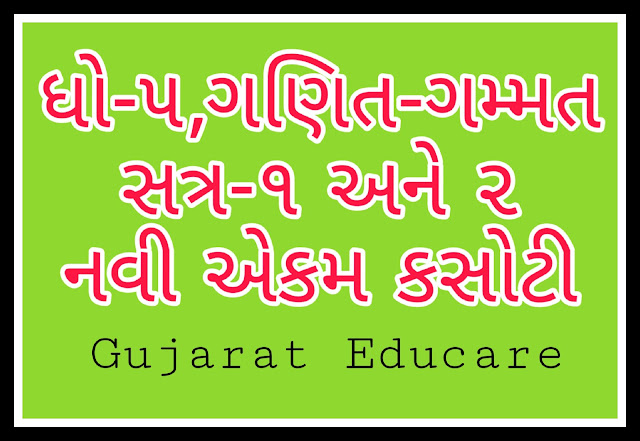# MATHEMATICS UNIT TEST : STANDARD - 5, SEMESTER - 1 & 2

MATHEMATICS UNIT TEST : STANDARD - 5, SEMESTER - 1 & 2

Mathematics is the study of such topics as quantity, structure,space and change.It has no generally accepted definition.Mathematicians seek and use patterns to formulate new conjectures; they resolve the truth or falsity of conjectures by mathematical proof. When mathematical structures are good models of real phenomena, then mathematical reasoning can provide insight or predictions about nature. Through the use of abstraction and logic, mathematics developed from counting, calculation, measurement, and the systematic study of the shapes and motions of physical objects. Practical mathematics has been a human activity from as far back as written records exist. The research required to solve mathematical problems can take years or even centuries of sustained inquiry.
GUJARATI ALL UNIT TESTS
Rigorous arguments first appeared in Greek mathematics, most notably in Euclid's Elements. Since the pioneering work of Giuseppe Peano (1858–1932), David Hilbert (1862–1943), and others on axiomatic systems in the late 19th century, it has become customary to view mathematical research as establishing truth by rigorous deduction from appropriately chosen axioms and definitions. Mathematics developed at a relatively slow pace until the Renaissance, when mathematical innovations interacting with new scientific discoveries led to a rapid increase in the rate of mathematical discovery that has continued to the present day.
SAUNI AASPAS ALL UNIT TESTS
Galileo Galilei (1564–1642) said, "The universe cannot be read until we have learned the language and become familiar with the characters in which it is written. It is written in mathematical language, and the letters are triangles, circles and other geometrical figures, without which means it is humanly impossible to comprehend a single word. Without these, one is wandering about in a dark labyrinth." Carl Friedrich Gauss (1777–1855) referred to mathematics as "the Queen of the Sciences". Benjamin Peirce (1809–1880) called mathematics "the science that draws necessary conclusions". David Hilbert said of mathematics: "We are not speaking here of arbitrariness in any sense. Mathematics is not like a game whose tasks are determined by arbitrarily stipulated rules. Rather, it is a conceptual system possessing internal necessity that can only be so and by no means otherwise." Albert Einstein(1879–1955) stated that "as far as the laws of mathematics refer to reality, they are not certain; and as far as they are certain, they do not refer to reality."
Mathematics is essential in many fields, including natural science, engineering, medicine, finance and the social sciences. Applied mathematics has led to entirely new mathematical disciplines, such as statistics and game theory. Mathematicians engage in pure mathematics, or mathematics for its own sake, without having any application in mind. Practical applications for what began as pure mathematics are often discovered.

FOR CHAPTER 1 & 2 : CLICK HEREFOR CHAPTER 3 TO 7 : CLICK HEREFOR SEM - 2 : CLICK HERERelated Posts

#### Tambahkan KomentarSembunyikan

Developed By Mukesh Galchar - The shixanpanth